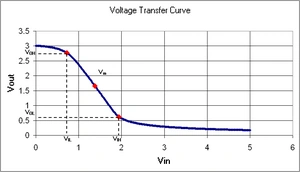## FANDOM

655 Pages

An inverter refers to two distinct types of electric circuits. In analog electronics, an inverter is a circuit for converting direct current to alternating current.

In digital electronics, an inverter is a circuit that converts a logic level 1 to a logic-level 0, and vice-versa.

## Analog invertersEdit

Analog inverters are used in a wide range of applications, from small power supplies for a computer to large industrial applications to transport bulk power. An inverter can have one or two switched-mode power supplies (SMPS).

Simple inverters consist of an oscillator driving a transistor that is used to interrupt the incoming direct current to create a square wave. This is then fed through a transformer to produce the required output voltage. Advanced inverters have started using more advanced forms of transistors or similar devices such as thyristors.

More efficient inverters use various tricks to try to get a reasonable sine wave at the transformer input, rather than relying on the transformer to smooth it. Capacitors and inductors can be used to smooth the flow of current into and out of the transistor. Also, it is possible to produce a more sinusoidal wave by having split-rail direct current inputs at two voltages, or positive and negative inputs with a central ground. By connecting the transformer input terminals in sequence between the positive rail and ground, the positive rail and the negative rail, the ground rail and the negative rail, then both to the ground rail, a stepped sinusoid is generated at the transformer input and the current drain on the direct current supply is less choppy. These methods result in an output that is called a "modified-sinewave". Modified-sine invertors may cause some loads, such as motors, to operate less efficiently.

More expensive power inverters use Pulse Width Modulation (PWM) with a high frequency carrier to more closely approximate a sine function. The quality of an inverter is described by its pulse-rating: a 3-pulse is a very simple arrangement, utilising only 3 transistors, whereas a more complex 12-pulse system will give an almost exact sine wave. In remote areas where a utility generated power is subject to significant external, distorting influences such as inductive loads or semiconductor-rectifier loads, a 12-pulse inverter may even offer a better, "cleaner" output than the utility-supplied power grid.

## Digital invertersEdit

Template:Mergeto A Digital Inverter is a circuit which outputs a voltage representing the opposite logic-level as its input. Digital electronics are circuits that operate at fixed voltage levels corresponding to a logical 0 or 1 (see Binary). An inverter circuit serves as the basic logic gate to swap between those two voltage levels. Implementation determines the actual voltage, but common levels include (0, +5V) for TTL circuits.

Common types include resistive-drain, using one transistor and one resistor; and CMOS, which uses two (opposite type) transistors per inverter circuit.

Digital inverter quality is often measured using the Voltage Transfer Curve, which is a plot of input vs. output voltage. From such a graph, device parameters including noise tolerance, gain, and operating logic-levels can be obtained.Voltage Transfer Curve for a 20 μm Inverter constructed at North Carolina State University

Ideally, the voltage transfer curve (VTC) appears as an inverted step-function - this would indicate precise switching between on and off - but in real devices, a gradual transition region exists. The VTC indicates that for low input voltage, the circuit outputs high voltage; for high input, the output tapers off towards 0 volts. The slope of this transition region is a measure of quality - steep (close to -Infinity) slopes yield precise switching.

The tolerance to noise can be measured by comparing the minimum input to the maximum output for each region of operation (on / off).

The output voltage VOH can be a measure of signal driving strength when cascading many devices together.

The digital inverter is considered the base building block for all digital electronics. Memory (1 bit register) is built as a Latch by feeding the output of two serial inverters together. Multiplexers, decoders, state machines, and other sophisticated digital devices all rely on the basic inverter.

## See alsoEdit

Simple inverters generate harmonics which affect the quality of power obtained using them. But PWM inverters eliminate this by means of a sine wave cancellation using the properties of Fourier Series.